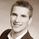จำนวนเข้าชม 4552
4552
For binary. Timeframe: 5 min.

Good binary broker: http://goo.gl/uGWMWb

P.S. thx ucsgears for idea
```study(title="StohDMI", shorttitle="StohDMI", overlay=false)

wwma(l,p) =>
wwma = (nz(wwma) * (l - 1) + p) / l

DMIlength = input(10, title = "Длина DMI")
Stolength = input(6, title = "Длина DMI Stochastic")
os = input (10, title = "Перепроданность")
ob = input (90, title = "Перекупленность")

hiDiff = high - high
loDiff = low - low

plusDM = (hiDiff > loDiff) and (hiDiff > 0) ? hiDiff : 0
minusDM = (loDiff > hiDiff) and (loDiff > 0) ? loDiff : 0

ATR = wwma(DMIlength, tr)

PlusDI = 100 * wwma(DMIlength,plusDM) / ATR
MinusDI = 100 * wwma(DMIlength,minusDM) / ATR

osc = PlusDI - MinusDI

hi = highest(osc, Stolength)
lo = lowest(osc, Stolength)

Stoch = sum((osc-lo),Stolength) / sum((hi-lo),Stolength) *100
plot(Stoch, color = black, title = 'Stochastic', linewidth = 2, style = line)

crossUp = Stoch < os and Stoch > os ? 1 : 0
crossDo = Stoch > ob and Stoch < ob ? 1 : 0

plot (ob, color = gray, linewidth = 1, title = 'Купить')
plot (os, color = gray, linewidth = 1, title = 'Продать')

plotchar(crossUp, title="Вверх", char='⇑', location=location.bottom, color=green, transp=0)
plotchar(crossDo, title="Вниз",char='⇓', location=location.top, color=red, transp=0)

line2 = hline(90, linestyle=dotted, color=black)
line1 = hline(45, color=black)
line0 = hline(10, linestyle=dotted, color=black)
fill(line2, line0)```Works very well with nadex platform for binary options
ตอบกลับfabfoot
Just add ", size=size.small)" behind "transp=0" in 36 and 37 line to:
ตอบกลับGood prediction... worked out well
ตอบกลับ